Next: Two-Dimensional Potential Flow Up: Two-Dimensional Incompressible Inviscid Flow Previous: Two-Dimensional Jets

# Exercises

1. For the case of the two-dimensional motion of an incompressible fluid, determine the condition that the velocity components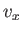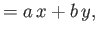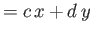satisfy the equation of continuity. Show that the magnitude of the vorticity is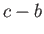.

2. For the case of the two-dimensional motion of an incompressible fluid, show that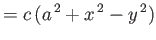are the velocity components of a possible flow pattern. Determine the stream function and sketch the streamlines. Prove that the motion is irrotational, and find the velocity potential.

3. A cylindrical vortex in an incompressible fluid is co-axial with the-axis, and such that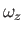takes the constant valuefor, and is zero for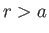, whereis a cylindrical coordinate. Show thatwhere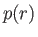is the pressure at radiusinside the vortex, and the circulation of the fluid outside the vortex is. Deduce thatwhere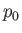is the pressure at the center of the vortex.

4. Consider the cylindrical vortex discussed in Exercise 3. Ifis the pressure at radiusexternal to the vortex, demonstrate that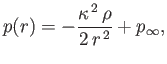whereis the pressure at infinity.

5. Show that the stream function for the cylindrical vortex discussed in Exercises 3 and 4 is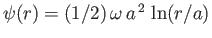for, andfor.

6. Prove that in the two-dimensional motion of a liquid the mean tangential fluid velocity around any small circle of radiusis, where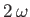is the value ofat the center of the circle. Neglect terms of order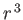.

7. Show that the equation of continuity for the two-dimensional motion of an incompressible fluid can be writtenwhere,are cylindrical coordinates. Demonstrate that this equation is satisfied when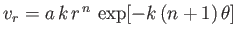and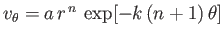. Determine the stream function, and show that the fluid speed at any point is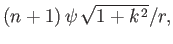where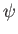is the stream function at that point (defined such that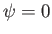at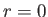).

8. Demonstrate that streamlines cross at right-angles at a stagnation point in two-dimensional, incompressible, irrotational flow.

9. Consider two-dimensional, incompressible, inviscid flow. Demonstrate that the fluid motion is governed by the following equations: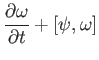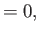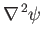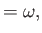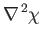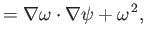where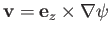,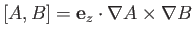, and.

10. For irrotational, incompressible, inviscid motion in two dimensions show that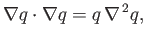where.Next: Two-Dimensional Potential Flow Up: Two-Dimensional Incompressible Inviscid Flow Previous: Two-Dimensional Jets
Richard Fitzpatrick 2016-03-31